# How does Magia DNI work

December 2011
Translated: October 2013
Updated: February 2017

© 2013, 2017 Josep Portella Florit

## Introduction

Magia DNI is an Android application that uses the device’s camera to read the OCR data of the DNI, the Spanish ID card, either the electronic or the traditional, and calculate the check digit, applying what’s explained in my article Demystifying the DNI numbers. The application’s purpose is to visually debunk the myth regarding the check digit, so I made it work even if the check digit is is covered.

After publishing Magia DNI several persons were interested in how I had done the character recognition. In this article I pretend to explain the method I used.

I didn’t use third-party libraries (if we don’t count Android’s SDK) but, to make it easier to implement, more precise and faster, I took advantage of the peculiarities of the OCR data of the DNI that generic OCR systems can’t consider.

Magia DNI’s source code is available under the GPL license.

## Character rows location

In Magia DNI the camera image is treated as a 2-dimensional matrix with values from 0 to 255 that represent each pixel’s luminosity, being 0 totally dark and 255 the maximum light value.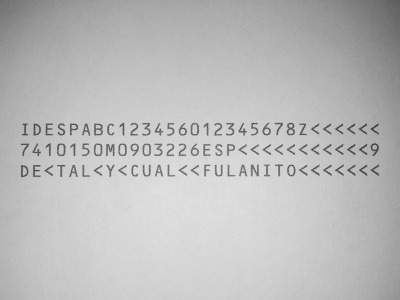The mean of luminosity is obtained for each horizontal line of pixels. The result of this process is a graph like this: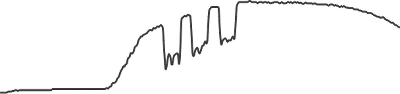Then, each value between the maximum and minimum of the graph is used as a threshold to calculate a collision graph. The collisions are a series of segments of which we know the position, length and the fact whether they’re above or below the threshold, i.e., if they collide or not collide with the graph. For example, setting the threshold between the maximum and minimum of the previous graph: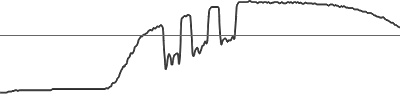this collision graph is obtained:The pattern left by the OCR data of the DNI is searched in each collision graph; if it is found, the rows positions are picked from the corresponding segments and are stored, but if a coincidence had already been found, this one is only replaced by the new one if it is better.

The pattern left by the DNI OCR data rows is three non-colliding segments, let’s call them rows, separated by smaller segments that collide, let’s call them separators. The rows are checked to be at least of a certain length, proportional to the size of the image, and the standard deviation of the length of the rows is checked to be less than the 12% of the mean of the lengths, and the same is done for the separators. Also, the maximum row length is checked to be greater than the maximum length of the separators, but less than the collisions on the left of the first row and on the right of the last row.

A pattern match is considered better than another when the sum of the lengths of its rows is greater than the other.

Once the process is done, the last row of the best match is discarded, since it has no use for the purpose of the application; the separators are also discarded, and the two remaining rows are adjusted to the left and to the right depending on their separation.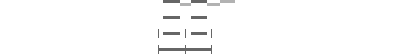This way the characters are less likely to be partially cut.

## Character columns location

Keeping in mind the position and the length of the two first rows, we see the image like this: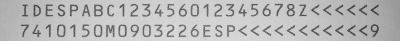For each vertical line of the cropped image, the maximum value and the minimum value are found and subtracted, resulting in a graph like this one: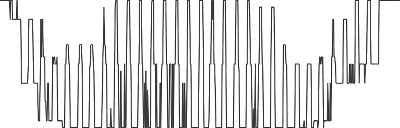As with the rows, for each value between the maximum and minimum of the graph a collision graph is obtained. For each collision graph, the columns pattern is searched and the best match is stored.

Let’s call columns the non colliding segments that match the position of the character columns. The pattern to be searched is similar to the rows pattern; the difference is that the maximum value of the standard deviation of the column lengths will be the mean of the lengths. It is considered correct if there are 24 columns (those needed to calculate the check digit), and like with the rows, the columns have certain minimum length and the maximum length of the columns is greater than the maximum length of the separators.

A match is considered better than another if the sum of the length of its columns is greater, and is placed on the same position or is leftmost.

Finally the separators of the best match are discarded and the columns are adjusted in the same way the rows were adjusted.

## Character 2-bit conversion

Having located the rows and the columns of characters, we already have the position, height and width of the characters we are interested in. Before being able to recognize the characters they have to be transformed from grayscale to 2-bit, to get rid of as much noise as possible. For this we calculate the optimal threshold: the 70% of the mean value of the pixels of the character to process.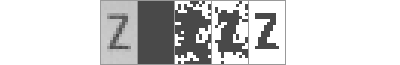## Character recognition

After knowing the optimal threshold of a character, we crop the margins to adjust it.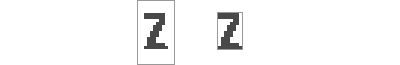When recognizing a character, the different possibilities depending on the row and column of the character are taken into account. For example, the dates on the second row will always be digits. This way the process is more precise and faster.

The application has a template for each possible character. For each possible character the image to recognize is compared with the corresponding template pixel by pixel, considering the ratio, since they won’t normally have the same size. In the beginning a template has the score set to zero. If a pixel matches, the score is increased; if it doesn’t match, it is decreased. The result will be the value of the template with the greatest score.

## DNI type recognition

The character on column 23 of the first row is recognized in order to know if the OCR data being read belongs to an electronic DNI or a traditional DNI. If the character is a digit, then it is an electronic DNI; if the character is a less-than symbol, then it is a traditional DNI.

The DNI type is needed when calculating the check digit, because the needed fields are different.

## Error detection

Before showing a calculated check digit, the other check digits are checked, including the DNI letter, to prevent showing the incorrect digit. However, 2 or more inopportune recognition errors could make it possible to overcome the tests, resulting in the wrong final check digit. To make this less provable, the digit is not shown unless the same digit is calculated for the next image, and it is discarded if it is a different digit.